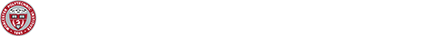# The Inverse First Passage Time Problem for killed Brownian motion

## The Inverse First Passage Time Problem for killed Brownian motion

Speaker: Alex Hening from Tufts

Date and Time: 10/08/19, 4-5pm

Abstract: The inverse ﬁrat passage time problem asks whether, for a Brownian motion B and a nonnegative random variable ζ, there exists a time-varying barrier b such that P{B s > b(s), 0 ≤ s ≤ t} = P{ζ > t}. We study a ”smoothed” version of this problem R t and ask whether there is a ”barrier” b such that E[exp(−λ ψ(B s − b(s)) ds)] = P{ζ > t}, 0 where λ is a killing rate parameter and ψ : R → [0, 1] is a non-increasing function. We prove that if ψ is suitably smooth, the function t → P{ζ > t} is twice continuously diﬀerentiable, and the condition 0 < − d log P{ζ>t}/dt < λ holds for the hazard rate of ζ, then there exists a dt unique continuously diﬀerentiable function b solving the smoothed problem. We show how this result leads to ﬂexible models of default for which it is possible to compute expected values of contingent claims.